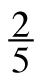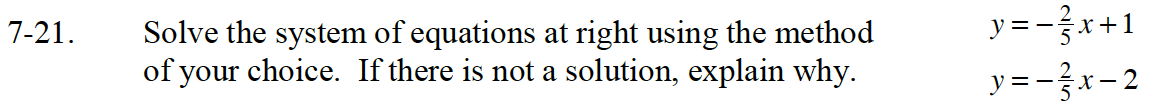Home > CC4 > Chapter 7 > Lesson 7.1.2 > Problem7-21

7-21.
1. Solve the system of equations below using the method of your choice. If there is not a solution, explain why. Homework Help ✎

y = −x + 1
y = −x – 2Which method of solving a system should be used here, Equal Values Method, Substitution, or Elimination?

This is a no solution. Why is there no solution? How can you tell by looking at the equations?